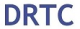# Rate of Change: Algebra

The rate of change, the rate of motion, the rate of a heartbeat. A chart on a piece of paper as boring as it may seem, can produce some pretty great numbers that directly relate to us human folk.

The question below may make you groan at first glance, but what happens if we use our imagination to picture the real-life bird it’s describing? Any better? Yes? No? Well, either way we must solve, so let’s get to it

## How do I answer this question?

It wants us to find the Rate of Change, specifically between 3 and 9 seconds. Let’s hi-light those two values on our given table:

## What is the Rate of Change?

To get the Rate of Change between these two values, we need to go back to the good ole’ Slope Formula and realize that the list of values is really a list of x and y coordinates.

Now let’s plug in the coordinate values (3, 6.26) and (9,3.41) into our slope formula:

Extra Tip! Notice that we added the labels feet/second to our answer.  Why does this make sense?? The question tells us that P(t) represents feet and that t is equal to seconds.  Another way to look at this question when applying it to the slope formula is to realize that we are finding the change of feet divided by the change of seconds.                                                          ____________________________________________________________________________________

Still got questions?  Let me know in the comments and as always happy calculating!:)

Looking for the next step? Learn how to graph equation of a line, y=mx+b here!

## 5 thoughts on “Rate of Change: Algebra”

1.oscarduyorjr says:

Thanks for the tip.